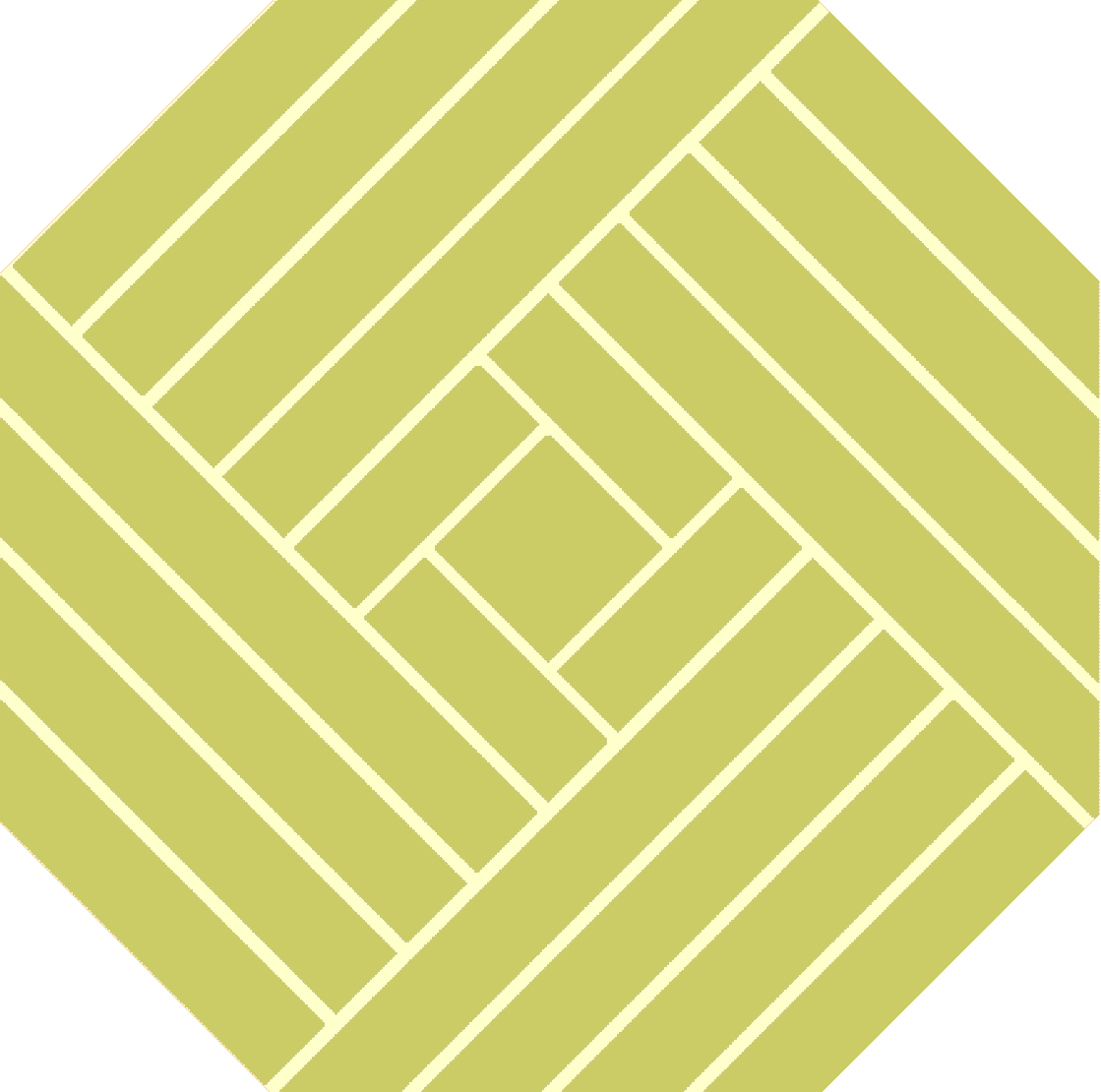## Belgian-Dutch Algebraic Geometry Day

### Award of the Compositio Mathematica PrizeThe 11th edition of this seminar will be held on Friday 9 December 2016, at the University of Amsterdam, Oudemanhuispoort, room D1.09. The speakers are the nominees for the Compositio Mathematica Prize, and the event will end with the award of the prize to Bhargav Bhatt.

This event is sponsored by the Foundation Compositio Mathematica.

### Programme

 13:30-14:30OMHP D1.09 June Huh (Princeton) Enumeration of points, lines, planes, etc. Abstract. One of the earliest results in enumerative combinatorial geometry is the following theorem of de Bruijn and Erdős: Every set of points E in a projective plane determines at least |E| lines, unless all the points are contained in a line. Motzkin and others extended the result to higher dimensions, who showed that every set of points E in a projective space determines at least |E| hyperplanes, unless all the points are contained in a hyperplane. Let E be a spanning subset of a d-dimensional vector space. We show that, in the poset of subspaces spanned by subsets of E, there are at least as many (d-k)-dimensional subspaces as there are k-dimensional subspaces, for every k at most d/2. This confirms the “top-heavy” conjecture of Dowling and Wilson from 1974 for all matroids realizable over some field. The proof relies on the decomposition theorem package for l-adic intersection complexes. Joint work with Botong Wang. 14:50-16:00OMHP D1.09 Dieter Kotschick (München) and Stefan Schreieder (Bonn) The Hodge ring of Kähler manifolds Abstract. In 1954, motivated by his proof of the Riemann-Roch theorem, Hirzebruch asked which combinations of Chern and Hodge numbers of smooth complex algebraic varieties are topological invariants of the underlying manifolds. Surprisingly, this question remained unanswered for almost sixty years. We shall describe a complete solution to this problem. This solution uses the Hodge ring, a natural object encoding the Hodge numbers of all compact Kähler manifolds. It follows from the structure of this ring that there are no unexpected relations among the Hodge numbers, and no essential differences between the Hodge numbers of smooth complex projective varieties and those of arbitrary Kähler manifolds. The consideration of certain natural ideals in the Hodge ring allows us to determine exactly which linear combinations of Hodge numbers are birationally invariant, and which are topological invariants 16:20-17:20OMHP D1.09 Bhargav Bhatt (Ann Arbor) Derived splinters in mixed characteristic Abstract. A derived splinter is a commutative ring that splits off (in the derived category) from the cohomology of any proper cover. Such rings are exactly rational singularities in characteristic 0 (Kovacs), and closely related to the analog in characteristic p. I will recall this story and its place in theory of F-singularities, and then explain why regular rings are derived splinters (de Jong's conjecture), even in mixed characteristic. The proof uses perfectoid techniques and builds on Andre's work on Hochster's direct summand conjecture. One of my main goals in this talk is to explain why passing from a mixed characteristic ring to a perfectoid extension is a useable analog of the passage to the perfection (direct limit over Frobenius) in characteristic p. 17:30-VOX-POP Award of Compositio Mathematica Prize and a reception at the Imaginary exhibition at VOX-POP (Binnengasthuisstraat 9, see this map).

### About the Belgian-Dutch Algebraic Geometry days

The Belgian-Dutch Algebraic Geometry days started in 2012, and takes place two to three times per year in Amsterdam, Leiden, Leuven, or Nijmegen. Previous editions: 1st, 2nd, 3rd, 4th, 5th, 6th, 7th, 8th, 9th, 10th.

The Algebraic Geometry days are organized by Nero Budur, Bas Edixhoven, Ben Moonen, Johannes Nicaise, and Lenny Taelman.